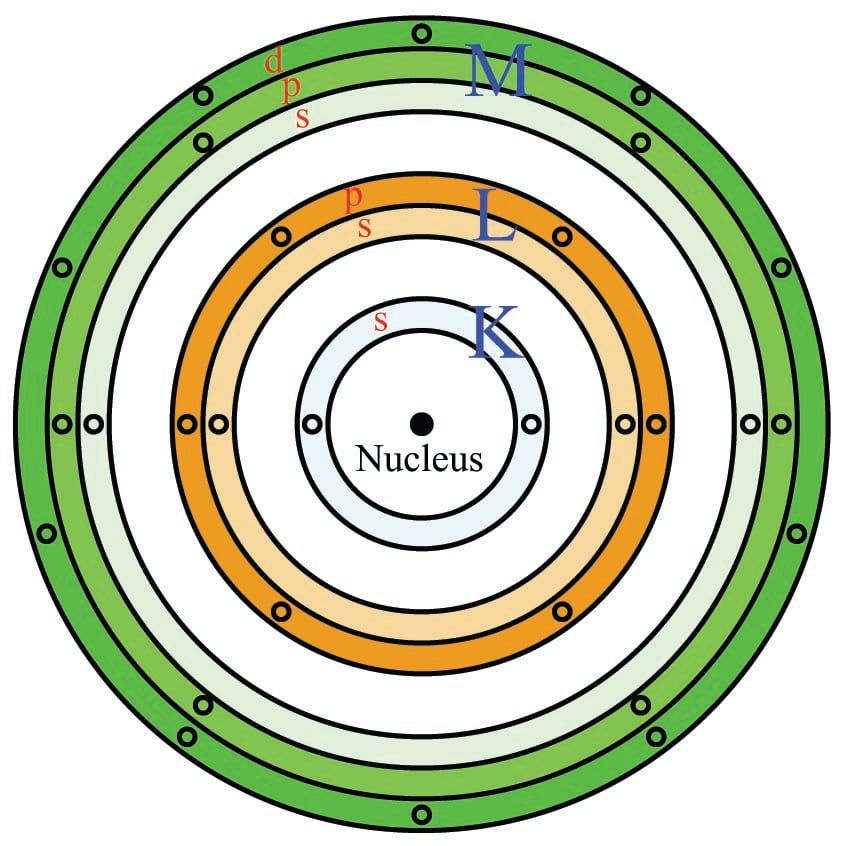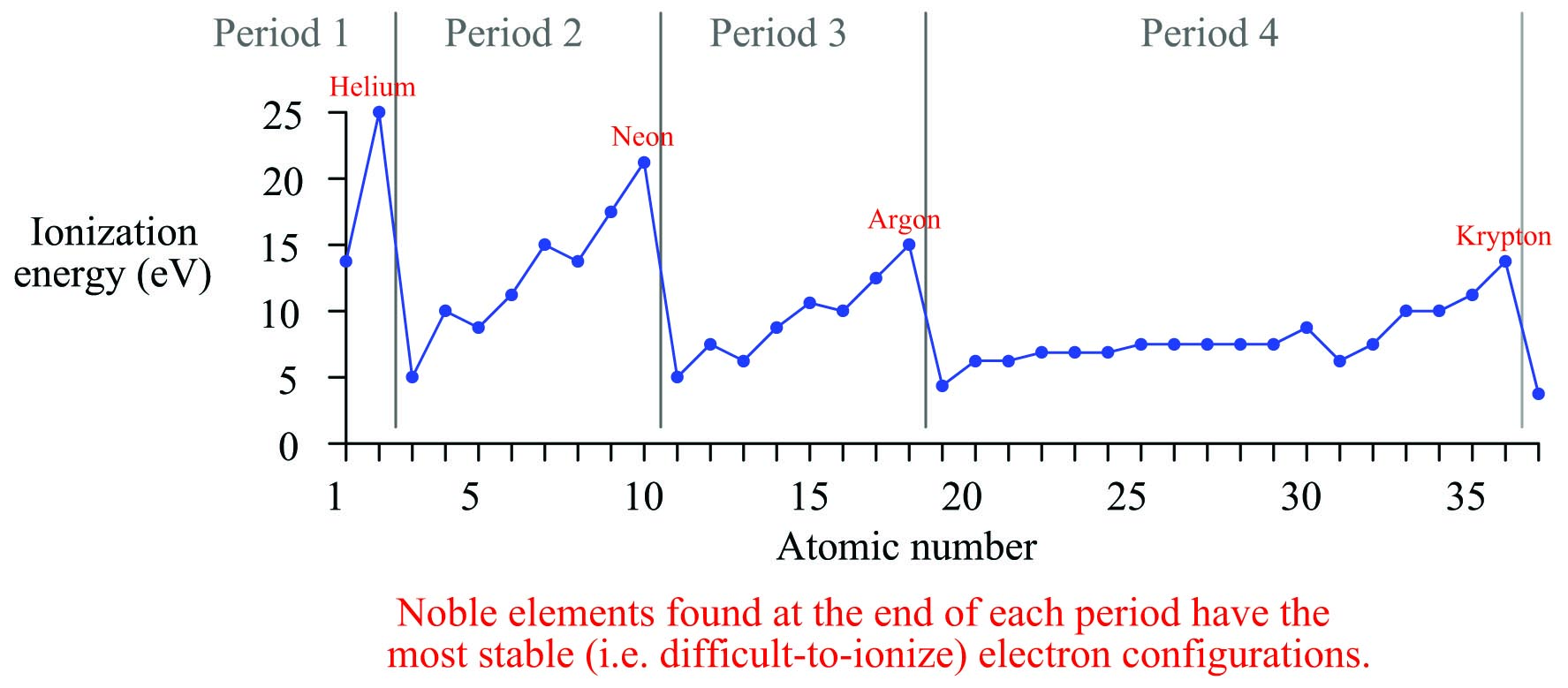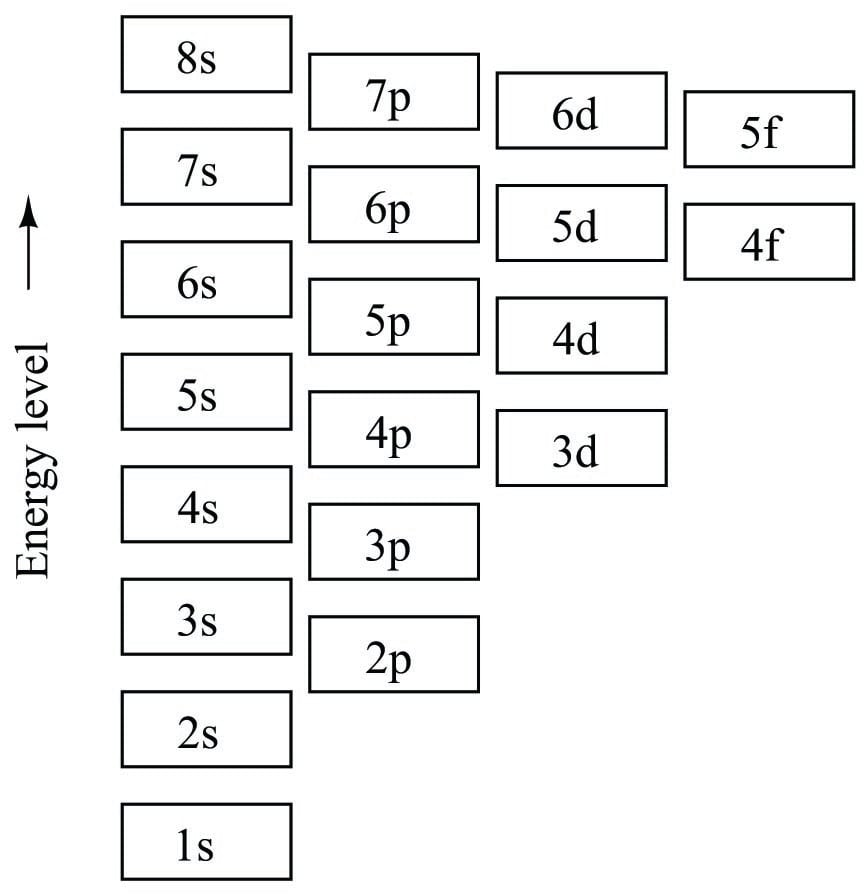Chapter Chemistry in Industrial Instrumentation

# Electronic Structure

Earlier in this chapter you were shown a model of a nitrogen atom with a dense nucleus (comprised of protons and neutrons) surrounded by electrons whirling around like satellites around a planet. While there are some useful features of this model, it is largely in error. A more realistic view of atomic structure begins with the realization that electrons do not exist as discrete particles, but rather as wave packets. In a sense, they orbit the nucleus within certain areas of probability, as described by the principles of quantum mechanics. One way to envision this is to think of an electron’s placement around the nucleus in the same way you might picture a city shrouded by a layer of fog. The electron does not have a discrete location (even though there is a discrete number of them found in every atom), but rather may be found anywhere within a certain region to varying degrees of probability.

Things get even stranger the more electrons there are in an atom. No two electrons may share the same quantum states in the same atom – a principle called the Pauli Exclusion Principle. This means the electrons surrounding a nucleus must exist in distinct patterns. Just a few of these patterns are shown here as orbitals (regions of high probability where up to two electrons may be found surrounding a nucleus):Electrons situate themselves around the nucleus of any atom according to one basic rule: the minimization of potential energy. That is, the electrons seek the lowest-energy positions available around the nucleus. Given the electrostatic attraction between negative electrons and the positive nucleus of an atom, there is potential energy stored in the “elevation” between an orbiting electron and the nucleus, just as there is gravitational potential energy in any object orbiting a planet. Electrons lose energy as they situate themselves closer to the nucleus, and it requires an external input of energy to move an electron farther away from its parent nucleus.

In a sense, most of chemistry may be explained by this principle of minimized potential energy. Electrons “want” to “fall” as close as they can to the positively-charged nucleus. However, there is limited “seating” around the nucleus. As described by Pauli’s Exclusion Principle, electrons cannot simply pile on top of each other in their quest for minimum energy, but rather must occupy certain regions of space allowed by their unique quantum states.

An analogy for visualizing this is to picture an atom as if it were an amphitheater, with the stage being the nucleus and the concentric array of seats being places where electrons may reside. All spectators (electrons) desire to be as close to the stage (nucleus) in an amphitheater (atom) as possible, but since everyone cannot occupy the best seat, people are forced to choose seats at different positions around the stage. As a result, the inner seats fill first, with most empty seats being furthest away from the stage. The concept of energy fits neatly into this analogy as well: just as electrons give up energy to “fall into” lower-energy regions around the nucleus, people must give up money to purchase seats closest to the action on stage.

The energy levels available for orbiting electrons are divided into categories of shells and subshells. A “shell” (or, principal quantum number, $$n$$) describes the main energy level of an electron. In our amphitheater analogy, this is equivalent to a tier or seating level. A “subshell” (or, subsidiary quantum number, $$l$$) further divides the energy levels within each electron shell, and assigns different shapes to the electrons’ probability “clouds.” In the amphitheater analogy, a subshell would be a row of seats within a particular tier. To make the analogy accurate, we would have to imagine each row of seats in a tier having a different shape (not all arcs or straight lines), with varying degrees of viewing comfort afforded by each shape. The first row in each tier faces uniformly toward the stage, allowing easy viewing. Successive rows (subshells) in each tier (shell) contain more seats, but are bent in such a way that the stage is not as easy to view, making these rows less desirable to occupy. Electron subshells always have an even-numbered electron capacity, analogous to theater rows containing even-numbered quantities of seats, because atomic electrons tend to gather in pairs called orbitals.

Chemists identify electron shells both by number (the value of the quantum number $$n$$) and/or by capital letters: the first shell by the letter K, the second by L, the third by M, and the fourth by N. Higher-order shells exist for atoms requiring many electrons (high atomic number), and the lettering pattern is alphabetic (fifth shell is O, sixth is P, etc.). Each successive shell has a greater number of subshells available, like successive amphitheater tiers having more rows: the low-level tiers closest to the stage having the fewest rows, and the high-level tiers furthest from the stage having the most rows.

A numbering and lettering system is also used by chemists to identify subshells within each shell (the $$l$$ quantum number value starting with zero, and lower-case letters beginning with “s”): the first subshell ($$l = 0$$) in any shell represented by the letter s, the second ($$l = 1$$) by p, the third ($$l = 2$$) by d, the fourth ($$l = 3$$) by f, and all others by successive lower-case letters of the alphabet. Each subshell of each shell has an even-numbered capacity for electrons, since the electrons in each subshell are organized in “orbital” regions, each orbital handling a maximum of two electrons. The number of orbitals per shell is equal to twice the $$l$$ value plus one. An “s” subshell has one orbital holding up to two electrons. A “p” subshell has three orbitals holding up to six electrons total. A “d” subshell has five orbitals holding up to ten electrons total. An “f” subshell has seven orbitals holding up to 14 electrons total. A “g” subshell has nine orbitals holding up to 18 electrons total.

The number of subshells in any shell is the same as that shell’s $$n$$ value. Thus, the first (K) shell has only one subshell, “s”. The second (L) shell has two subshells, an “s” and a “p”. The third (M) shell has three subshells available, an “s”, a “p”, and a “d”; and so on.

This table shows the first few shells, their subshells, and electron capacities of each:

($n$ value) & ($l$ value) & $=2(2l + 1)$ \cr \noalign{\hrule \vskip 10pt \hrule} \noalign{\hrule \vskip 10pt \hrule} \noalign{\hrule \vskip 10pt \hrule} \noalign{\hrule \vskip 10pt \hrule}

 Shell Subshell Subshell electron capacity $n = 1$ ; K $l = 0$ ; s $2 \times (2 \times 0 + 1) = 2$ $n = 2$ ; L $l = 0$ ; s $2 \times (2 \times 0 + 1) = 2$ $l = 1$ ; p $2 \times (2 \times 1 + 1) = 6$ $n = 3$ ; M $l = 0$ ; s $2 \times (2 \times 0 + 1) = 2$ $l = 1$ ; p $2 \times (2 \times 1 + 1) = 6$ $l = 2$ ; d $2 \times (2 \times 2 + 1) = 10$ $n = 4$ ; N $l = 0$ ; s $2 \times (2 \times 0 + 1) = 2$ $l = 1$ ; p $2 \times (2 \times 1 + 1) = 6$ $l = 2$ ; d $2 \times (2 \times 2 + 1) = 10$ $l = 3$ ; f $2 \times (2 \times 3 + 1) = 14$

Reviewing our amphitheater analogy, atomic shells are like seating tiers (levels), subshells are like rows of seats within each tier, and subshell electron capacity is like the number of seats in each row. This simple illustration shows an atom with three shells (K, L, and M) with the respective subshells (s, p, and d) represented by differently-shaded rings within each shell, having different numbers of places for electrons within each one:This illustration is vastly over-simplified, failing to show the diverse shapes of each subshell, serving only to show how each successive shell grows in subshells and electron capacities.

The complete electron configuration for an atom may be expressed using spectroscopic notation, showing the shell numbers, subshell letters, and number of electrons residing within each subshell as a superscript. For example, the element helium (with an atomic number of 2) would be expressed as 1s$$^{2}$$, with just two electrons in the “s” subshell of the first shell. The following table shows the electron structures of the first nineteen elements in the periodic table, from the element hydrogen (atomic number = 1) to potassium (atomic number = 19):

Element Atomic number Electron configuration
Hydrogen 1 1s$^{1}$
Helium 2 1s$^{2}$
Lithium 3 1s$^{2}$2s$^{1}$
Beryllium 4 1s$^{2}$2s$^{2}$
Boron 5 1s$^{2}$2s$^{2}$2p$^{1}$
Carbon 6 1s$^{2}$2s$^{2}$2p$^{2}$
Nitrogen 7 1s$^{2}$2s$^{2}$2p$^{3}$
Oxygen 8 1s$^{2}$2s$^{2}$2p$^{4}$
Fluorine 9 1s$^{2}$2s$^{2}$2p$^{5}$
Neon 10 1s$^{2}$2s$^{2}$2p$^{6}$
Sodium 11 1s$^{2}$2s$^{2}$2p$^{6}$3s$^{1}$
Magnesium 12 1s$^{2}$2s$^{2}$2p$^{6}$3s$^{2}$
Aluminum 13 1s$^{2}$2s$^{2}$2p$^{6}$3s$^{2}$3p$^{1}$
Silicon 14 1s$^{2}$2s$^{2}$2p$^{6}$3s$^{2}$3p$^{2}$
Phosphorus 15 1s$^{2}$2s$^{2}$2p$^{6}$3s$^{2}$3p$^{3}$
Sulfur 16 1s$^{2}$2s$^{2}$2p$^{6}$3s$^{2}$3p$^{4}$
Chlorine 17 1s$^{2}$2s$^{2}$2p$^{6}$3s$^{2}$3p$^{5}$
Argon 18 1s$^{2}$2s$^{2}$2p$^{6}$3s$^{2}$3p$^{6}$
Potassium 19 1s$^{2}$2s$^{2}$2p$^{6}$3s$^{2}$3p$^{6}$4s$^{1}$

In order to avoid having to write unwieldy spectroscopic descriptions of each element’s electron structure, it is customary to write the notation only for subshells that are unfilled. For example, instead of writing the electron structure of the element aluminum as 1s$$^{2}$$2s$$^{2}$$2p$$^{6}$$3s$$^{2}$$3p$$^{1}$$, we might just as well write a condensed version showing only the last subshell (3p$$^{1}$$), since all the previous subshells are completely full.

Another way to abbreviate the spectroscopic notation for elements is to condense all the shells below the newest (unfilled) shell as the corresponding noble element, in brackets. To use the example of aluminum again, we could write its spectroscopic notation as [Ne]3s$$^{2}$$3p$$^{1}$$ since its shell 1 and shell 2 configurations are completely described by the electron configuration of Neon.

Re-writing our electron shell table for the first nineteen elements using this condensed notation:

Element Atomic number Electron configuration
Hydrogen 1 1s$^{1}$
Helium 2 1s$^{2}$
Lithium 3 [He]2s$^{1}$
Beryllium 4 [He]2s$^{2}$
Boron 5 [He]2s$^{2}$2p$^{1}$
Carbon 6 [He]2s$^{2}$2p$^{2}$
Nitrogen 7 [He]2s$^{2}$2p$^{3}$
Oxygen 8 [He]2s$^{2}$2p$^{4}$
Fluorine 9 [He]2s$^{2}$2p$^{5}$
Neon 10 [He]2s$^{2}$2p$^{6}$
Sodium 11 [Ne]3s$^{1}$
Magnesium 12 [Ne]3s$^{2}$
Aluminum 13 [Ne]3s$^{2}$3p$^{1}$
Silicon 14 [Ne]3s$^{2}$3p$^{2}$
Phosphorus 15 [Ne]3s$^{2}$3p$^{3}$
Sulfur 16 [Ne]3s$^{2}$3p$^{4}$
Chlorine 17 [Ne]3s$^{2}$3p$^{5}$
Argon 18 [Ne]3s$^{2}$3p$^{6}$
Potassium 19 [Ar]4s$^{1}$

If we progress from element to element in increasing atomic number, we see that no new shell begins to form until after we reach the noble element for that period at the far right-hand column. With the beginning of each new period at the far-left end of the Table, we see the beginning of the next higher-order electron shell. The shell(s) below are represented by whichever noble element shares that same configuration, indicating a “noble core” of electrons residing in extremely stable (low-energy) regions around the nucleus.

The beginning of the next higher-order shell is what accounts for the periodic cycle of ionization energies we see in elements of progressing atomic number. The first electron to take residence in a new shell is very easy to remove, unlike the electrons residing in the “noble” configuration shell(s) below:Not only is the “noble core” notation convenient for tersely describing the electron structure of an element, but it also reveals an important concept in chemistry: the idea of valence. Electrons residing in lower-order shells are, by definition, at lower energy states than electrons residing in higher-order shells and are therefore much more difficult to dislodge. Therefore, the electrons in unfilled shells, being easier to dislodge, play a far greater role in chemical bonds than electrons residing in filled shells below. These “outer” electrons are called valence electrons, and their number determines how readily an atom will chemically interact with another atom. This is why elements found in the same group (having similar outer-electron configurations) bear similar chemical characteristics: the electrons lying below in the “noble core” configurations have little effect on how the atom will bond with other atoms. A lithium atom, with its outer-most electron configuration being 2s$$^{1}$$, reacts in much the same way as an atom of sodium having an outer-most configuration of 3s$$^{1}$$, and much the same as a potassium atom having an outer-most configuration of 4s$$^{1}$$, all because those outer-shell electrons are the most available for interaction with electrons of other atoms.

If we examine the electron structures of atoms with successively greater atomic numbers (more protons in the nucleus, therefore more electrons in orbit to balance the electrical charge), we notice that the shells and subshells fill up in an interesting pattern. One might think that all the lower-order shells get completely filled before any electrons go into a higher-order shell – just as we might expect people to fill every seat in all the lower tiers of an amphitheater before filling seats in any of the higher tiers – but this is not always the case. Instead, the energy levels of subshells within shells is split, such that certain subshells within a higher shell will have a lower energy value than certain subshells within a lower shell. Referring back to our amphitheater analogy, where seating tiers represented shells and seat rows of various shape represented subshells, it is as though people choose to fill the more comfortable rows in higher-level tiers before sitting in the less-comfortable rows in lowest available tiers, the desire for comfort trumping the desire for proximity to the stage.

A rule commonly taught in introductory chemistry courses called the Madelung rule (also referred to as Aufbau order, after the German verb aufbauen meaning “to build up”) is that subshells fill with increasing atomic number in such an order that the subshell with the lowest $$n + l$$ value, in the lowest shell, gets filled before any others.

The following graphic illustrates this ordering:Madelung filling order: 1s $$\rightarrow$$ 2s $$\rightarrow$$ 2p $$\rightarrow$$ 3s $$\rightarrow$$ 3p $$\rightarrow$$ 4s $$\rightarrow$$ 3d $$\rightarrow$$ 4p $$\rightarrow$$ 5s $$\rightarrow$$ 4d $$\rightarrow$$ 5p $$\rightarrow$$ 6s $$\rightarrow$$ 4f $$\rightarrow$$ 5d $$\rightarrow$$ 6p $$\rightarrow$$ 7s $$\rightarrow$$ 5f $$\rightarrow$$ 6d $$\rightarrow$$ 7p $$\rightarrow$$ 8s $$\rightarrow$$ (etc.)

It should be noted that exceptions exist for this rule. We see one of those exceptions with the element chromium ($$_{24}$$Cr). Strictly following the Madelung rule in progressing from vanadium (atomic number = 23, valence electron structure 3d$$^{3}$$4s$$^{2}$$) to chromium (atomic number = 24), we would expect the next electron to take residence in the “3d” subshell making chromium’s valence structure be 3d$$^{4}$$4s$$^{2}$$, but instead we find two more electrons residing in chromium’s 3d subshell with one less in the 4s subshell (3d$$^{5}$$4s$$^{1}$$). The sequence resumes its expected progression with the next element, manganese (atomic number = 25, valence electron structure 3d$$^{5}$$4s$$^{2}$$). The general principle of energy minimization still holds true . . . it’s just that the relative energies of succeeding subshells do not follow a simple rule structure. In other words, the Aufbau order is an over-simplified view of reality. To use the amphitheater analogy again, it’s as if someone gave up one of the nice chairs in tier 4 to sit next to a friend who just occupied one of the less comfortable chairs in tier 3.

The practical importance of electron configurations in chemistry is the potential energy possessed by electrons as they reside in different shells and subshells. This is extremely important in the formation and breaking of chemical bonds, which occur due to the interaction of electrons between two or more atoms. A chemical bond occurs between atoms when the outer-most (valence) electrons of those atoms mutually arrange themselves in energy states that are collectively lower than they would be individually. The ability for different atoms to join in chemical bonds completely depends upon the default energy states of electrons in each atom, as well as the next available energy states in the other atoms. Atoms will form stable bonds only if the union allows electrons to assume stable (low-energy) levels. This is why different elements are very selective regarding which elements they will chemically bond with to form compounds: not all combinations of atoms result in favorable potential energy levels.

The amount of energy required to break a chemical bond (i.e. separate the atoms from each other) is the same amount of energy required to restore the atoms’ electrons to their previous (default) states before they joined. This is the same amount of energy released by the atoms as they come together to form the bond. Thus, we see the foundation of the general principle in chemistry that forming chemical bonds releases energy, while breaking chemical bonds requires an input of energy from an external source. We also see in this fact an expression of the Conservation of Energy: the amount of energy “invested” in breaking bonds is precisely the same as the amount of energy “returned” when those same bonds re-form.

In summary, the whole of chemistry is a consequence of electrons not being able to assume arbitrary positions around the nucleus of an atom. Instead, they seek the lowest possible energy levels within a framework allowing them to retain unique quantum states. Atoms with mutually agreeable electron structures readily bond together to form molecules, and they release energy in the process of joining. Molecules may be broken up into their constituent atoms, if sufficient energy is applied to overcome the bond. Atoms with incompatible electron structures do not form stable bonds with each other.

#### Lessons in Industrial Automation

Published under the terms and conditions of the Creative Commons Attribution 4.0 International Public License